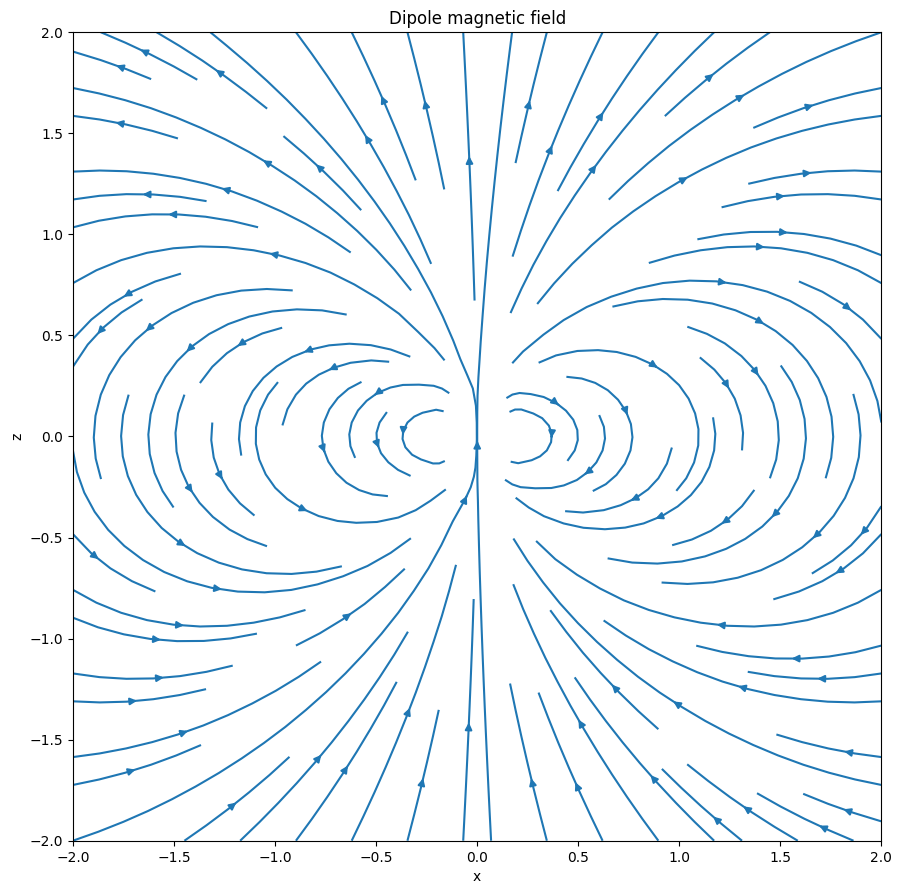# Magnetostatics (plasmapy.formulary.magnetostatics)

Define MagneticStatics class to calculate common static magnetic fields as first raised in issue #100.

## Classes

 CircularWire(normal, center, radius, current) Circular wire(coil) class. FiniteStraightWire(p1, p2, current) Finite length straight wire class. GeneralWire(parametric_eq, t1, t2, current) General wire class described by its parametric vector equation InfiniteStraightWire(direction, p0, current) Infinite straight wire class. MagneticDipole(moment, p0) Simple magnetic dipole — two nearby opposite point charges. Abstract class for all kinds of magnetic static fields Abstract wire class for concrete wires to be inherited from.## Examples